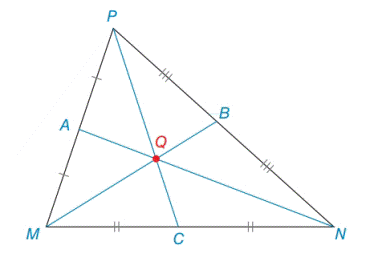Chapter 7.2, Problem 27EElementary Geometry For College St...

7th Edition
Alexander + 2 others
ISBN: 9781337614085

Solutions

Chapter
SectionElementary Geometry For College St...

7th Edition
Alexander + 2 others
ISBN: 9781337614085
Textbook Problem

In Δ M N P , medians M B ¯ , N A ¯ and P C ¯ intersect at centroid Q .a) If M Q = 8 , find Q B .b) If Q C = 3 , find P Q .c) If A Q = 3.5 , find A N .Exercises 27, 28

To determine

(a)

To find:

The QB in the given triangle MNP.

Explanation

Definition:

The three medians of a triangle are congruent at a point that is two-thirds the distance from any vertex to the midpoint of the opposite side.

Given:

In ΔMNP, medians MB¯, NA¯ and PC¯ intersect at centroid Q. If MQ=8.

Calculation:

The point of concurrence Q for the three medians of a triangle is known as centroid of the triangle. In ΔMNP, medians MB¯, NA¯ and PC¯ intersect at centroid Q

To determine

(b)

To find:

The PQ in the given triangle MNP.

To determine

(c)

To find:

The AN in the given triangle MNP.

Still sussing out bartleby?

Check out a sample textbook solution.

See a sample solution

The Solution to Your Study Problems

Bartleby provides explanations to thousands of textbook problems written by our experts, many with advanced degrees!

Get Started

Find more solutions based on key concepts